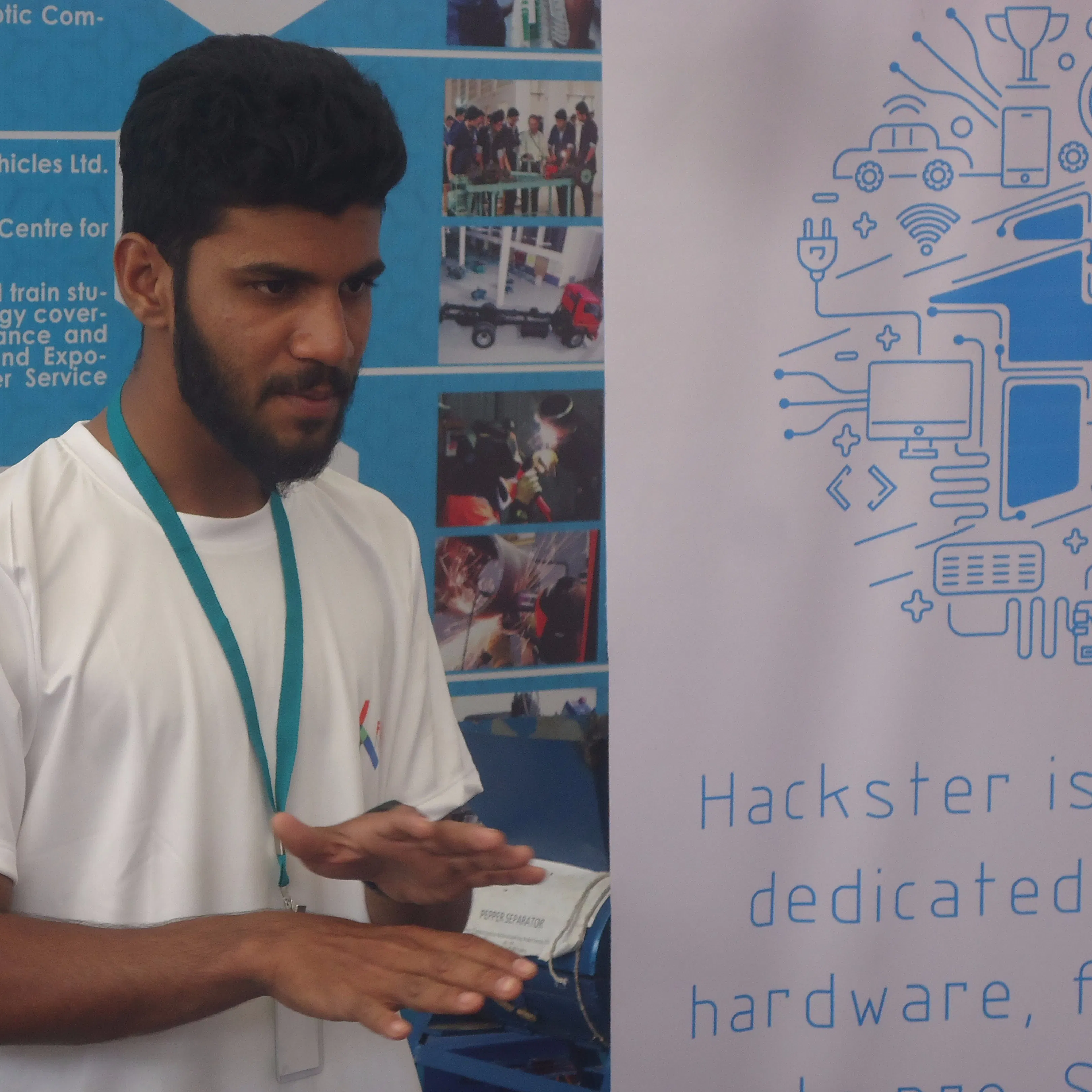# Malaria Detection using Open Microscope and Deep learning

An open-source microscope that can detect disease like malaria, the main goal is to give quality health checkup to poor people.

## Things used in this project

### Hardware componentsNVIDIA Jetson Nano Developer Kit
×1Raspberry Pi Camera Module
×1High Brightness LED, White
×1USB-A to Mini-USB Cable
×1
×1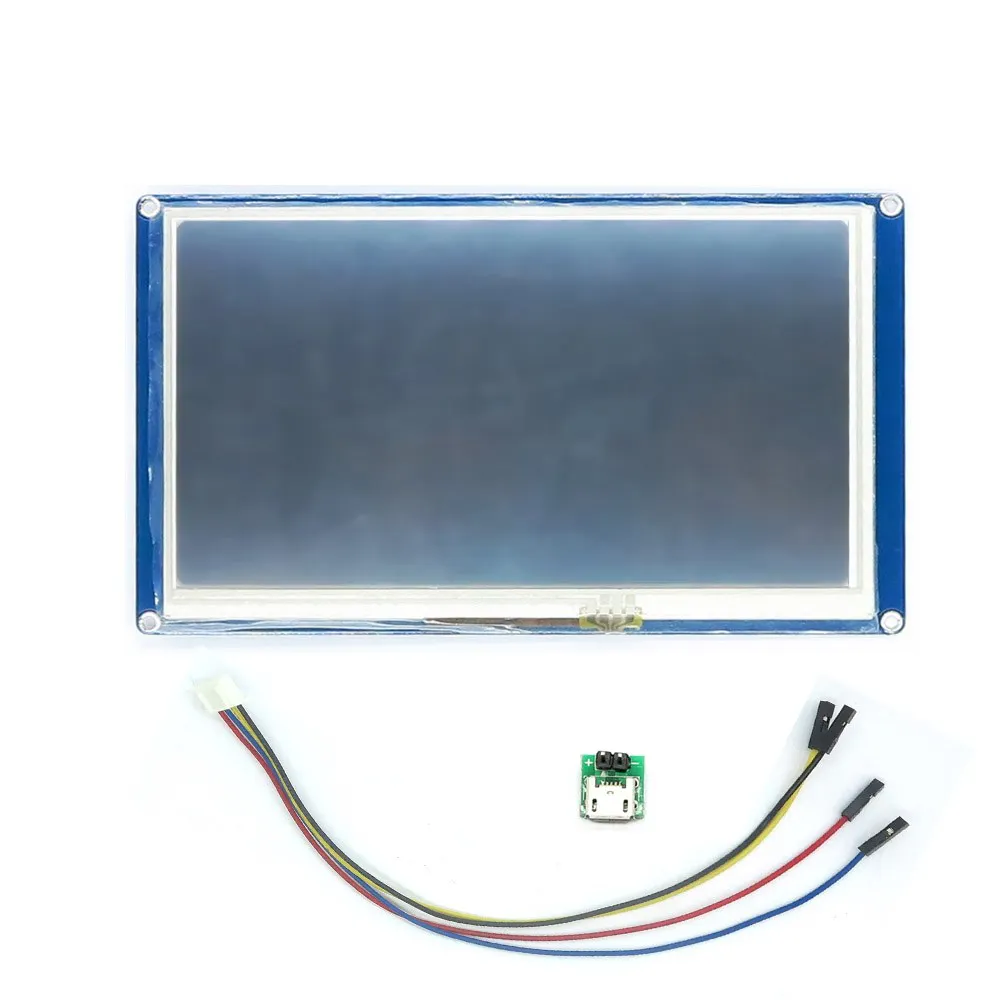Itead Nextion NX8048T070 - Generic 7.0" HMI TFT LCD Touch Display
×1

### Software apps and online services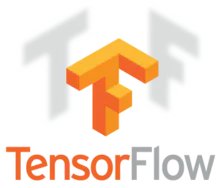TensorFlow
 Jetpack SDK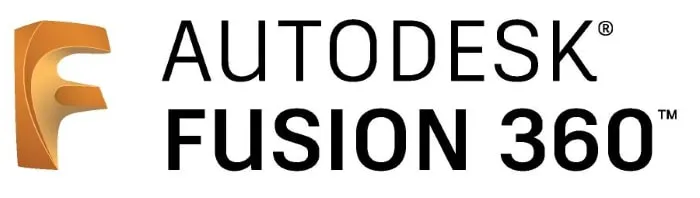Autodesk Fusion 360

### Hand tools and fabrication machines3D Printer (generic)

## Schematics

### Uninfected and Parasitized Model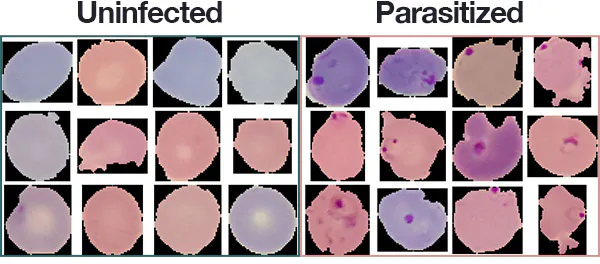### Blood cells using Open Microscope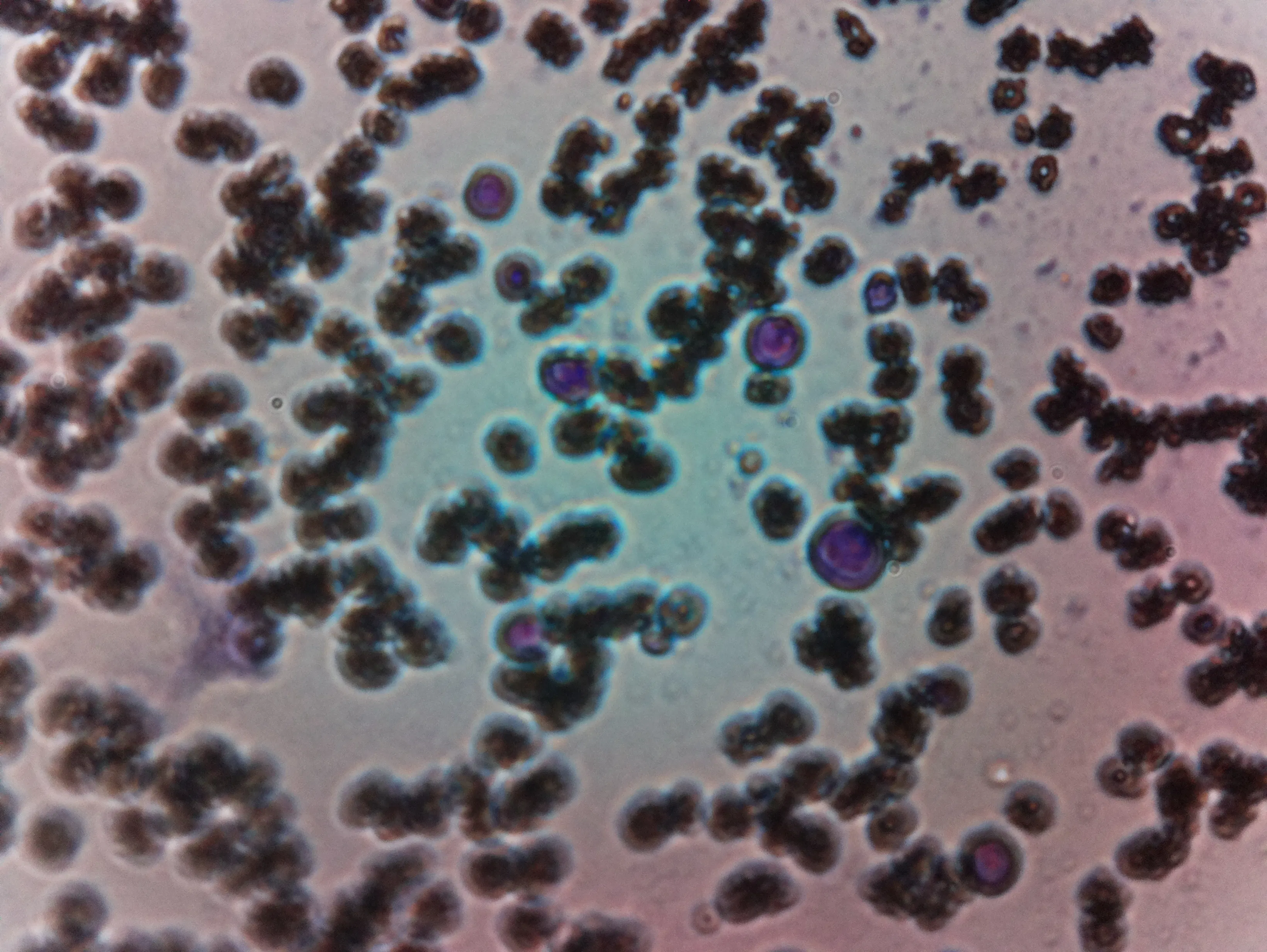### Proposed Solution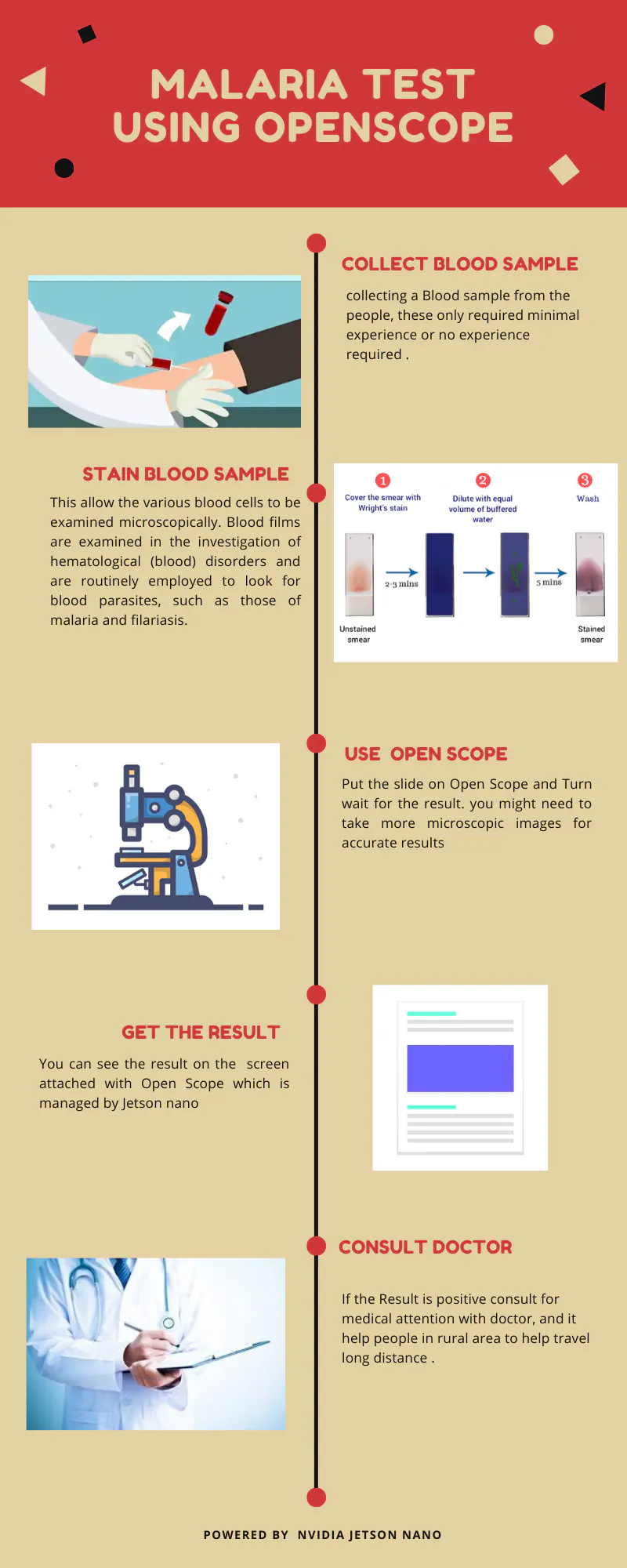## Code

### save_model.py

Python
```# USAGE
# python save_model.py --dataset malaria --model saved_model.model

# set the matplotlib backend so figures can be saved in the background
import matplotlib
matplotlib.use("Agg")

# import the necessary packages
from keras.preprocessing.image import ImageDataGenerator
from keras.optimizers import SGD
from pyimagesearch.resnet import ResNet
from sklearn.metrics import classification_report
from imutils import paths
import matplotlib.pyplot as plt
import numpy as np
import argparse
import os

# construct the argument parser and parse the arguments
ap = argparse.ArgumentParser()
help="path dataset of input images")
help="path to trained model")
help="path to output loss/accuracy plot")
args = vars(ap.parse_args())

# initialize the number of training epochs and batch size
NUM_EPOCHS = 25
BS = 32

# derive the path to the directories containing the training,
# validation, and testing splits, respectively
TRAIN_PATH = os.path.sep.join([args["dataset"], "training"])
VAL_PATH = os.path.sep.join([args["dataset"], "validation"])
TEST_PATH = os.path.sep.join([args["dataset"], "testing"])

# determine the total number of image paths in training, validation,
# and testing directories
totalTrain = len(list(paths.list_images(TRAIN_PATH)))
totalVal = len(list(paths.list_images(VAL_PATH)))
totalTest = len(list(paths.list_images(TEST_PATH)))

# initialize the training training data augmentation object
trainAug = ImageDataGenerator(
rescale=1 / 255.0,
rotation_range=20,
zoom_range=0.05,
width_shift_range=0.05,
height_shift_range=0.05,
shear_range=0.05,
horizontal_flip=True,
fill_mode="nearest")

# initialize the validation (and testing) data augmentation object
valAug = ImageDataGenerator(rescale=1 / 255.0)

# initialize the training generator
trainGen = trainAug.flow_from_directory(
TRAIN_PATH,
class_mode="categorical",
target_size=(64, 64),
color_mode="rgb",
shuffle=True,
batch_size=32)

# initialize the validation generator
valGen = valAug.flow_from_directory(
VAL_PATH,
class_mode="categorical",
target_size=(64, 64),
color_mode="rgb",
shuffle=False,
batch_size=BS)

# initialize the testing generator
testGen = valAug.flow_from_directory(
TEST_PATH,
class_mode="categorical",
target_size=(64, 64),
color_mode="rgb",
shuffle=False,
batch_size=BS)

# initialize our Keras implementation of ResNet model and compile it
model = ResNet.build(64, 64, 3, 2, (2, 2, 3),
(32, 64, 128, 256), reg=0.0005)
opt = SGD(lr=1e-1, momentum=0.9, decay=1e-1 / NUM_EPOCHS)
model.compile(loss="binary_crossentropy", optimizer=opt,
metrics=["accuracy"])

# train our Keras model
H = model.fit_generator(
trainGen,
steps_per_epoch=totalTrain // BS,
validation_data=valGen,
validation_steps=totalVal // BS,
epochs=NUM_EPOCHS)

# reset the testing generator and then use our trained model to
# make predictions on the data
print("[INFO] evaluating network...")
testGen.reset()
predIdxs = model.predict_generator(testGen,
steps=(totalTest // BS) + 1)

# for each image in the testing set we need to find the index of the
# label with corresponding largest predicted probability
predIdxs = np.argmax(predIdxs, axis=1)

# show a nicely formatted classification report
print(classification_report(testGen.classes, predIdxs,
target_names=testGen.class_indices.keys()))

# save the network to disk
print("[INFO] serializing network to '{}'...".format(args["model"]))
model.save(args["model"])

# plot the training loss and accuracy
N = NUM_EPOCHS
plt.style.use("ggplot")
plt.figure()
plt.plot(np.arange(0, N), H.history["loss"], label="train_loss")
plt.plot(np.arange(0, N), H.history["val_loss"], label="val_loss")
plt.plot(np.arange(0, N), H.history["acc"], label="train_acc")
plt.plot(np.arange(0, N), H.history["val_acc"], label="val_acc")
plt.title("Training Loss and Accuracy on Dataset")
plt.xlabel("Epoch #")
plt.ylabel("Loss/Accuracy")
plt.legend(loc="lower left")
plt.savefig(args["plot"])
```

### train_model.py

Python
```# USAGE
# python train_model.py

# set the matplotlib backend so figures can be saved in the background
import matplotlib
matplotlib.use("Agg")

# import the necessary packages
from keras.preprocessing.image import ImageDataGenerator
from keras.callbacks import LearningRateScheduler
from keras.optimizers import SGD
from pyimagesearch.resnet import ResNet
from pyimagesearch import config
from sklearn.metrics import classification_report
from imutils import paths
import matplotlib.pyplot as plt
import numpy as np
import argparse

# construct the argument parser and parse the arguments
ap = argparse.ArgumentParser()
help="path to output loss/accuracy plot")
args = vars(ap.parse_args())

# define the total number of epochs to train for along with the
# initial learning rate and batch size
NUM_EPOCHS = 20
INIT_LR = 1e-1
BS = 32

def poly_decay(epoch):
# initialize the maximum number of epochs, base learning rate,
# and power of the polynomial
maxEpochs = NUM_EPOCHS
baseLR = INIT_LR
power = 1.0

# compute the new learning rate based on polynomial decay
alpha = baseLR * (1 - (epoch / float(maxEpochs))) ** power

# return the new learning rate
return alpha

# determine the total number of image paths in training, validation,
# and testing directories
totalTrain = len(list(paths.list_images(config.TRAIN_PATH)))
totalVal = len(list(paths.list_images(config.VAL_PATH)))
totalTest = len(list(paths.list_images(config.TEST_PATH)))

# initialize the training training data augmentation object
trainAug = ImageDataGenerator(
rescale=1 / 255.0,
rotation_range=20,
zoom_range=0.05,
width_shift_range=0.05,
height_shift_range=0.05,
shear_range=0.05,
horizontal_flip=True,
fill_mode="nearest")

# initialize the validation (and testing) data augmentation object
valAug = ImageDataGenerator(rescale=1 / 255.0)

# initialize the training generator
trainGen = trainAug.flow_from_directory(
config.TRAIN_PATH,
class_mode="categorical",
target_size=(64, 64),
color_mode="rgb",
shuffle=True,
batch_size=BS)

# initialize the validation generator
valGen = valAug.flow_from_directory(
config.VAL_PATH,
class_mode="categorical",
target_size=(64, 64),
color_mode="rgb",
shuffle=False,
batch_size=BS)

# initialize the testing generator
testGen = valAug.flow_from_directory(
config.TEST_PATH,
class_mode="categorical",
target_size=(64, 64),
color_mode="rgb",
shuffle=False,
batch_size=BS)

# initialize our ResNet model and compile it
model = ResNet.build(64, 64, 3, 2, (3, 4, 6),
(64, 128, 256, 512), reg=0.0005)
opt = SGD(lr=INIT_LR, momentum=0.9)
model.compile(loss="binary_crossentropy", optimizer=opt,
metrics=["accuracy"])

# define our set of callbacks and fit the model
callbacks = [LearningRateScheduler(poly_decay)]
H = model.fit_generator(
trainGen,
steps_per_epoch=totalTrain // BS,
validation_data=valGen,
validation_steps=totalVal // BS,
epochs=NUM_EPOCHS,
callbacks=callbacks)

# reset the testing generator and then use our trained model to
# make predictions on the data
print("[INFO] evaluating network...")
testGen.reset()
predIdxs = model.predict_generator(testGen,
steps=(totalTest // BS) + 1)

# for each image in the testing set we need to find the index of the
# label with corresponding largest predicted probability
predIdxs = np.argmax(predIdxs, axis=1)

# show a nicely formatted classification report
print(classification_report(testGen.classes, predIdxs,
target_names=testGen.class_indices.keys()))

# plot the training loss and accuracy
N = NUM_EPOCHS
plt.style.use("ggplot")
plt.figure()
plt.plot(np.arange(0, N), H.history["loss"], label="train_loss")
plt.plot(np.arange(0, N), H.history["val_loss"], label="val_loss")
plt.plot(np.arange(0, N), H.history["acc"], label="train_acc")
plt.plot(np.arange(0, N), H.history["val_acc"], label="val_acc")
plt.title("Training Loss and Accuracy on Dataset")
plt.xlabel("Epoch #")
plt.ylabel("Loss/Accuracy")
plt.legend(loc="lower left")
plt.savefig(args["plot"])
```

### build_dataset.py

Python
```# USAGE
# python build_dataset.py

# import the necessary packages
from pyimagesearch import config
from imutils import paths
import random
import shutil
import os

# grab the paths to all input images in the original input directory
# and shuffle them
imagePaths = list(paths.list_images(config.ORIG_INPUT_DATASET))
random.seed(42)
random.shuffle(imagePaths)

# compute the training and testing split
i = int(len(imagePaths) * config.TRAIN_SPLIT)
trainPaths = imagePaths[:i]
testPaths = imagePaths[i:]

# we'll be using part of the training data for validation
i = int(len(trainPaths) * config.VAL_SPLIT)
valPaths = trainPaths[:i]
trainPaths = trainPaths[i:]

# define the datasets that we'll be building
datasets = [
("training", trainPaths, config.TRAIN_PATH),
("validation", valPaths, config.VAL_PATH),
("testing", testPaths, config.TEST_PATH)
]

# loop over the datasets
for (dType, imagePaths, baseOutput) in datasets:
# show which data split we are creating
print("[INFO] building '{}' split".format(dType))

# if the output base output directory does not exist, create it
if not os.path.exists(baseOutput):
print("[INFO] 'creating {}' directory".format(baseOutput))
os.makedirs(baseOutput)

# loop over the input image paths
for inputPath in imagePaths:
# extract the filename of the input image along with its
# corresponding class label
filename = inputPath.split(os.path.sep)[-1]
label = inputPath.split(os.path.sep)[-2]

# build the path to the label directory
labelPath = os.path.sep.join([baseOutput, label])

# if the label output directory does not exist, create it
if not os.path.exists(labelPath):
print("[INFO] 'creating {}' directory".format(labelPath))
os.makedirs(labelPath)

# construct the path to the destination image and then copy
# the image itself
p = os.path.sep.join([labelPath, filename])
shutil.copy2(inputPath, p)
```

### Malaria Model

XML
Keras models
`No preview (download only).`

Python
```# USAGE
# python load_model.py --images malaria/testing --model saved_model.model

# import the necessary packages
from keras.preprocessing.image import img_to_array
from imutils import build_montages
from imutils import paths
import numpy as np
import argparse
import random
import cv2

# construct the argument parser and parse the arguments
ap = argparse.ArgumentParser()
help="path to out input directory of images")
help="path to pre-trained model")
args = vars(ap.parse_args())

# grab all image paths in the input directory and randomly sample them
imagePaths = list(paths.list_images(args["images"]))
random.shuffle(imagePaths)
imagePaths = imagePaths[:16]

# initialize our list of results
results = []

# loop over our sampled image paths
for p in imagePaths:
# load our original input image

# pre-process our image by converting it from BGR to RGB channel
# ordering (since our Keras mdoel was trained on RGB ordering),
# resize it to 64x64 pixels, and then scale the pixel intensities
# to the range [0, 1]
image = cv2.cvtColor(orig, cv2.COLOR_BGR2RGB)
image = cv2.resize(image, (64, 64))
image = image.astype("float") / 255.0

# order channel dimensions (channels-first or channels-last)
# depending on our Keras backend, then add a batch dimension to
# the image
image = img_to_array(image)
image = np.expand_dims(image, axis=0)

# make predictions on the input image
pred = model.predict(image)
pred = pred.argmax(axis=1)

# an index of zero is the 'parasitized' label while an index of
# one is the 'uninfected' label
label = "Parasitized" if pred == 0 else "Uninfected"
color = (0, 0, 255) if pred == 0 else (0, 255, 0)

# resize our original input (so we can better visualize it) and
# then draw the label on the image
orig = cv2.resize(orig, (128, 128))
cv2.putText(orig, label, (3, 20), cv2.FONT_HERSHEY_SIMPLEX, 0.5,
color, 2)

# add the output image to our list of results
results.append(orig)

# create a montage using 128x128 "tiles" with 4 rows and 4 columns
montage = build_montages(results, (128, 128), (4, 4))

# show the output montage
cv2.imshow("Results", montage)
cv2.waitKey(0)
```

## Credits

### Salman Faris

16 projects • 320 followers
Maker | Hardware Hacker | Electronics Enthusiast